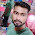### Program 177:To Calculate Permutation value nPr

Program 177:

```#include<stdio.h>
int factorial(int);
main()
{
int n,r;
float nPr;
printf("Enter value of n in nPr\n");
scanf("%d",&n);
printf("Enter value of r in nPr\n");
scanf("%d",&r);
if(n>=r&&n>=0&&r>=0)
{
nPr=(float)factorial(n)/factorial(n-r);
printf("Value of %dP%d=%f\n",n,r,nPr);
}
else
{
printf("Invalid Entry\n");
}

}

int factorial(int num)
{
int i,fact=1;
for(i=1;i<=num;i++)
{
fact=fact*i;
}

return(fact);
}
```
Explanation:
Formula for permutation us nPr=n!/(n-r)! under condition of  n>=r,n>=0,r>=0

`int factorial(int); → function Declaration`
1. Here we used
• n → To store Value
• r → To store Value
• nPr → To store output
2. ```printf("Enter value of n in nPr\n");
scanf("%d",&n);
printf("Enter value of r in nPr\n");
scanf("%d",&r);```
Takes values of n and r
3.
```if(n>=r&&n>=0&&r>=0)
{
nPr=(float)factorial(n)/factorial(n-r);
printf("Value of %dP%d=%f\n",n,r,nPr);
}
else
{
printf("Invalid Entry\n");
}```
Checks the required condition as mentioned in the above formula.If the condition is satisfied then if part is executed otherwise the else part.
4. ```nPr=(float)factorial(n)/factorial(n-r);
printf("Value of %dP%d=%f\n",n,r,nPr);```
As per formula we need to get factorial of 'n' and 'n-r'.factorial (n) gets the factorial of n same as factorial(n-r).As the factorial(n) send the value of n to factorial function .
```int factorial(int num)
{
int i,fact=1;
for(i=1;i<=num;i++)
{
fact=fact*i;
}

return(fact);
}```
After getting the value from factorial it calculates formula and store in nPr.

Output:

#### 1 comment:

1.factorial hundred In the last few days, the “factorial of 100” is one of the top subjects and a lot of maths geeks compute it using voice assistants such as Alexa, Shiri, etc.
factorial-of-hundred In the last few days, the “factorial of 100” is one of the top subjects and a lot of maths geeks compute it using voice assistants such as Alexa, Shiri, etc.

 Donate Buy me a coffee \$2.00 USD Buy me a burger \$5.00 USD Buy me a pizza \$10.00 USDCompiler Used by me C-Free 5.0(Recommended) Other Compilers Code Blocks(Recommended) Online Compilers Ideone(Remember to give input before executing online where ever necessary)